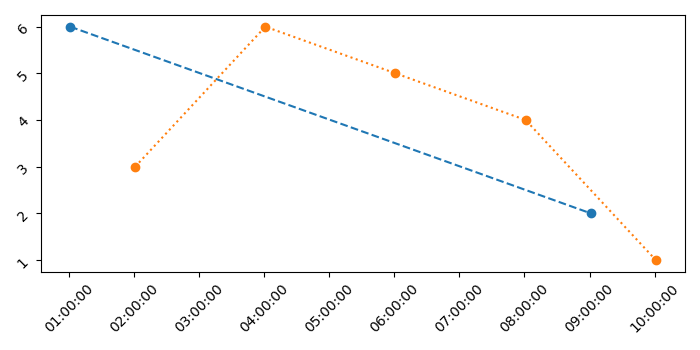# How can I plot two different spaced time series on one same plot in Python Matplotlib?

To plot two different spaced time series on one same plot using Matplotlib, we can take the following steps

## Steps

• Set the figure size and adjust the padding between and around the subplots.

• Create x1, y1 and x2 and y2 data points.

• Create a figure and a set of subplots.

• Plot the data that contains dates, with (x1, y1) and (x2, y2) data points.

• Set the major formatter of the X-axis ticklabels.

• Rotate xtick label by 45 degrees using tick_params() method.

• To display the figure, use Show() method.

## Example

import matplotlib.pyplot as plt
from matplotlib.dates import date2num, DateFormatter
import datetime as dt

plt.rcParams["figure.figsize"] = [7.00, 3.50]
plt.rcParams["figure.autolayout"] = True

x1 = [date2num(dt.datetime(2021, 1, 15, 1, 1, 1)), date2num(dt.datetime(2021, 1, 15, 9, 1, 1))]

y1 = [6, 2]

x2 = [ date2num(dt.datetime(2021, 1, 15, 2, 1, 1)),
date2num(dt.datetime(2021, 1, 15, 4, 1, 1)),
date2num(dt.datetime(2021, 1, 15, 6, 1, 1)),
date2num(dt.datetime(2021, 1, 15, 8, 1, 1)),
date2num(dt.datetime(2021, 1, 15, 10, 1, 1))
]

y2 = [3, 6, 5, 4, 1]

fig, ax = plt.subplots()

ax.plot_date(x1, y1, 'o--')
ax.plot_date(x2, y2, 'o:')

ax.xaxis.set_major_formatter(DateFormatter('%H:%M:%S'))
ax.tick_params(rotation=45)

plt.show()


## Output

It will produce the following output −